# Find the volume of the ice cream cone that is bounded above by the half sphere...

Find the volume of the ice cream cone that is bounded above by the half sphere z = sqrt( 4− x^2 − y^2 ) + 4 and the cone z= 2sqrt( x^2 + y^2 ). Transformation to cylindrical coordinates could be easier.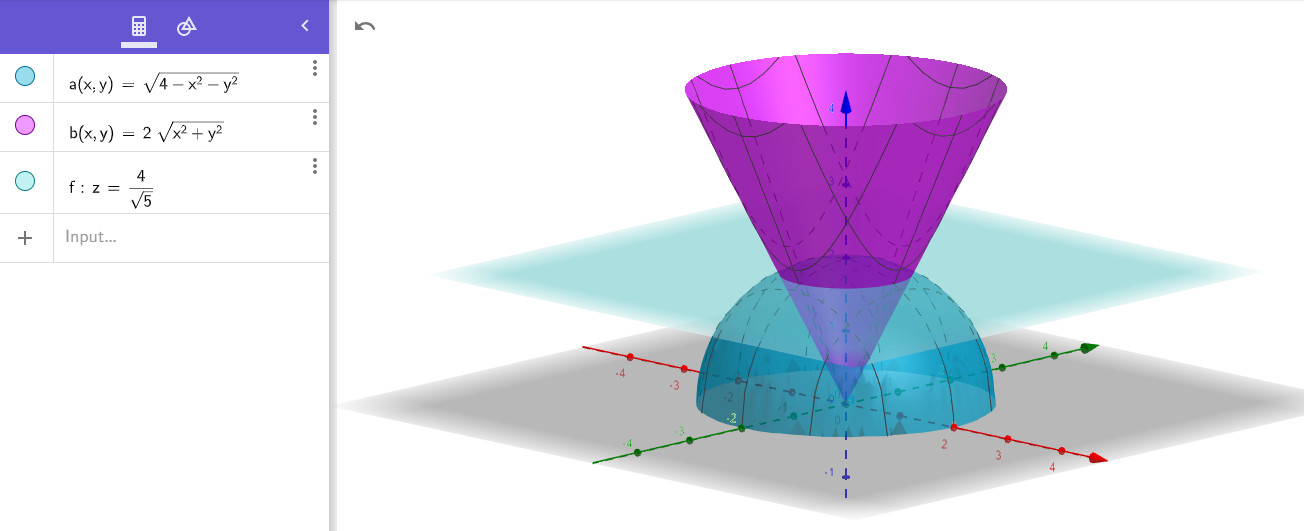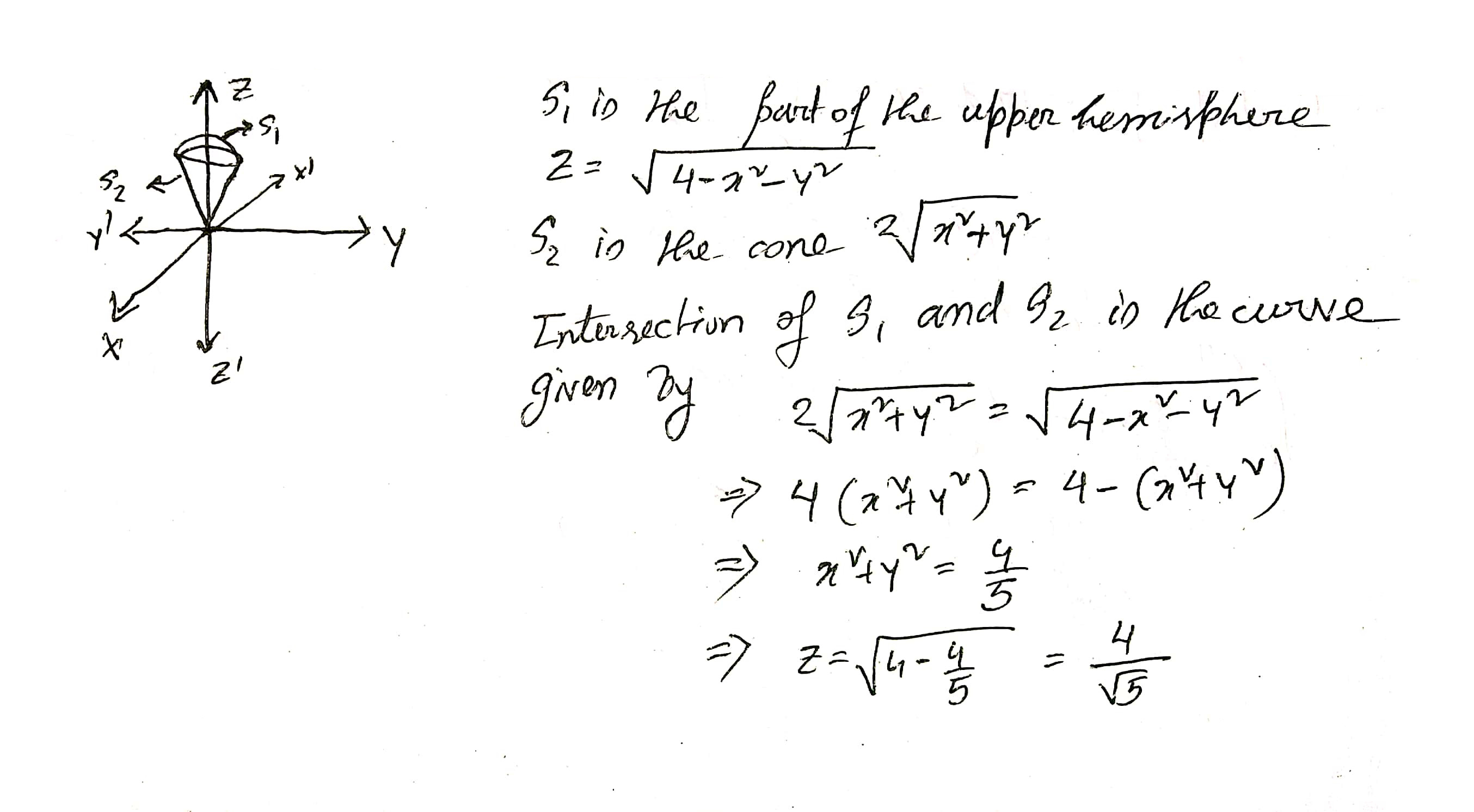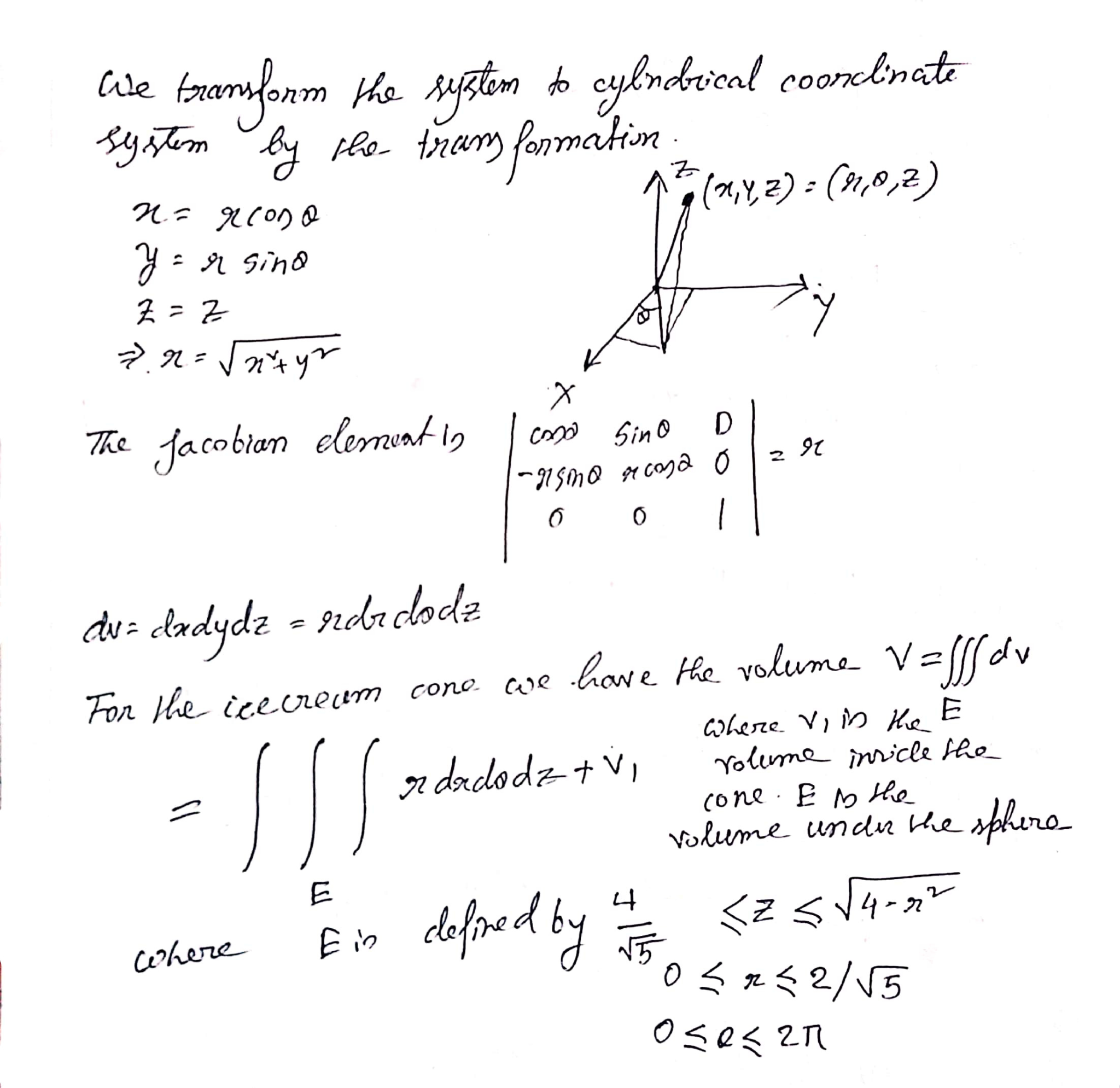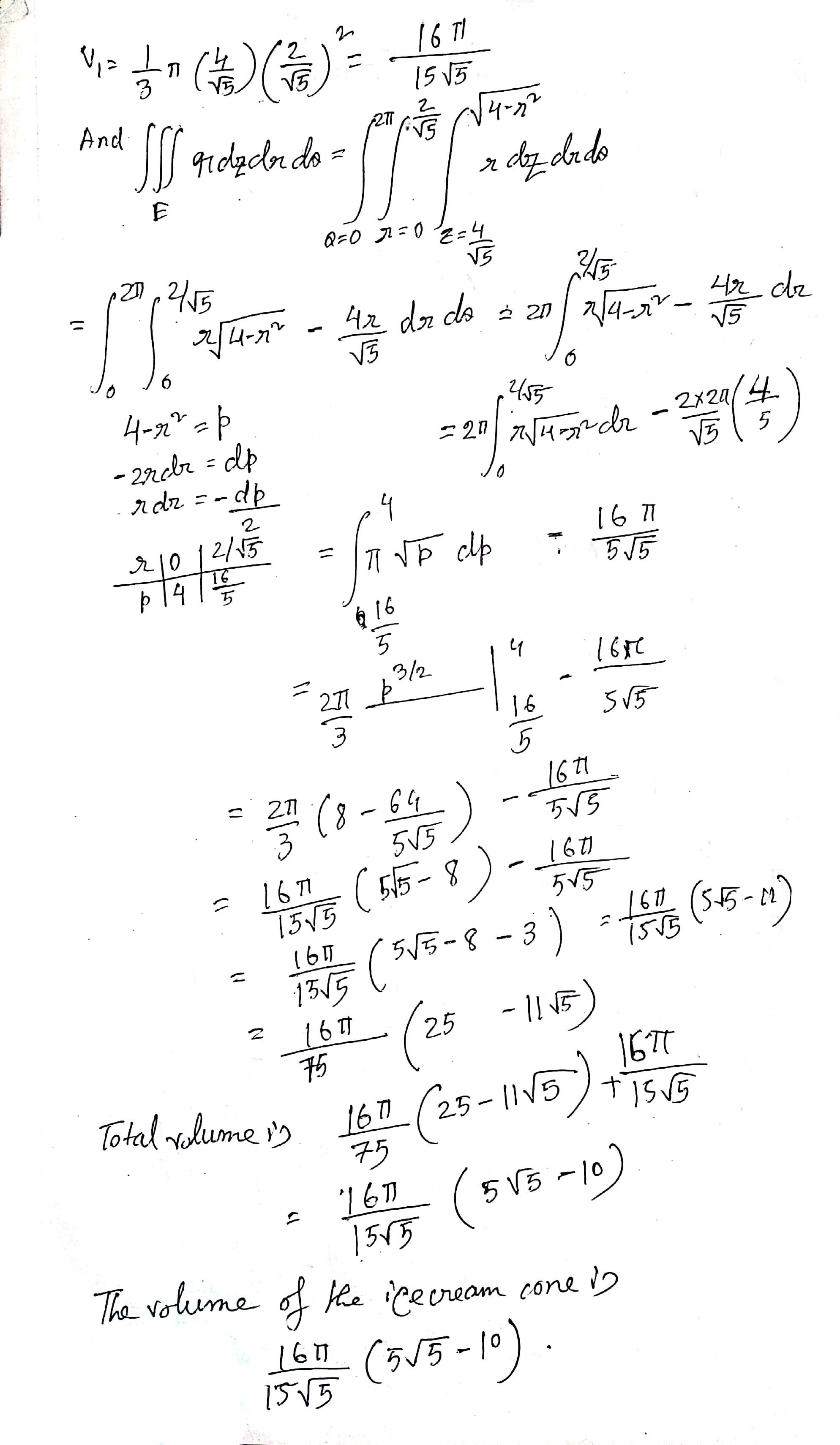##### Add Answer of: Find the volume of the ice cream cone that is bounded above by the half sphere...
Similar Homework Help Questions
• ### Problem 2 Let D be the "ice cream cone" region in space, bounded below by 2y3+y) and above by the...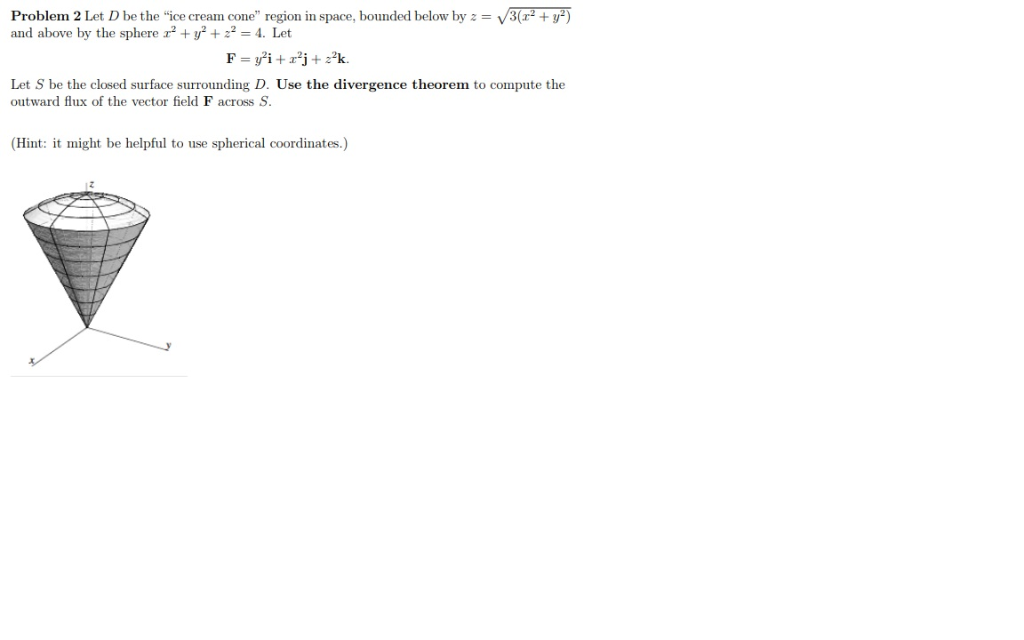Problem 2 Let D be the "ice cream cone" region in space, bounded below by 2y3+y) and above by the sphere 2y224. Let Let S be the closed surface surrounding D. Use the divergence theorem to compute the outward flux of the vector field F across S (Hint: it might be helpful to use spherical coordinates.) Problem 2 Let D be the "ice cream cone" region in space, bounded below by 2y3+y) and above by the sphere 2y224. Let Let...

• ### (a) Let R be the solid in the first octant which is bounded above by the sphere 22 + y2+2 2 and bounded below by the cone z- r2+ y2. Sketch a diagram of intersection of the solid with the rz plane...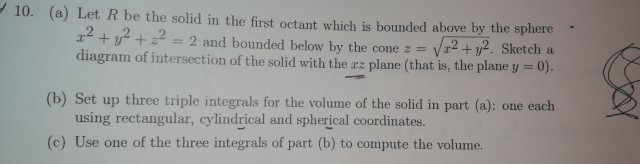(a) Let R be the solid in the first octant which is bounded above by the sphere 22 + y2+2 2 and bounded below by the cone z- r2+ y2. Sketch a diagram of intersection of the solid with the rz plane (that is, the plane y 0). / 10. (b) Set up three triple integrals for the volume of the solid in part (a): one each using rectangular, cylindrical and spherical coordinates. (c) Use one of the three integrals...

• ### Find volume of solid bounded by cone and sphere

Find the volume of the solid bounded by the cone z = √(3x2 + 3y2) and the sphere x2 + y2 + z2 = 82.

• ### Surface Area of sphere above cone

Find the surface area of the part of the sphere x^2+y^2+z^2=64 that lies above the cone z=sqrt(x^2+y^2).

• ### Exercise 6.3: Let U be the solid bounded below by the cone : _V3z? + 3y2 and above by the sphere ...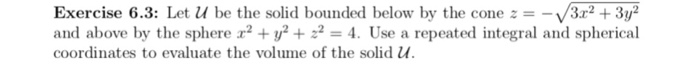Exercise 6.3: Let U be the solid bounded below by the cone : _V3z? + 3y2 and above by the sphere x2 + y2 + ~2 4. Use a repeated integral and spherical coordinates to evaluate the volume of the solid U Exercise 6.3: Let U be the solid bounded below by the cone : _V3z? + 3y2 and above by the sphere x2 + y2 + ~2 4. Use a repeated integral and spherical coordinates to evaluate the volume...

• ### Integration with polar coordinates

How do you solve this?Consider the solid shaped like an ice cream cone that is bounded by the functions z = Sqrt(x^2+y^2) and z = Sqrt(32-x^2-y^2) Set up an integral in polar coordinates tofind the volume of this ice cream cone.Please show the steps, especially for the bounds.

• ### (a) Find the volume of the region bounded above by the sphere x2 +y2 +z225 and below by the plane z - 4 by using cylind...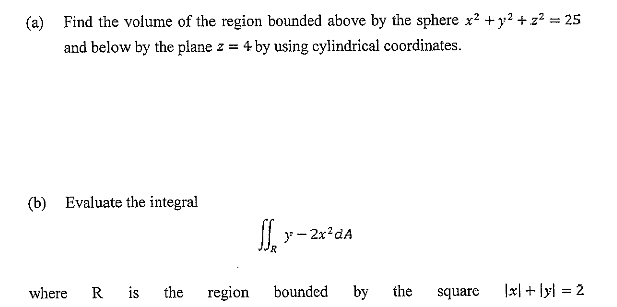(a) Find the volume of the region bounded above by the sphere x2 +y2 +z225 and below by the plane z - 4 by using cylindrical coordinates Evaluate the integral (b) 2x2dA ER where R is the region bounded by the square - 2 Show transcribed image text

• ### Find the volume of the solid Use spherical coordinates to find the mass of the solid...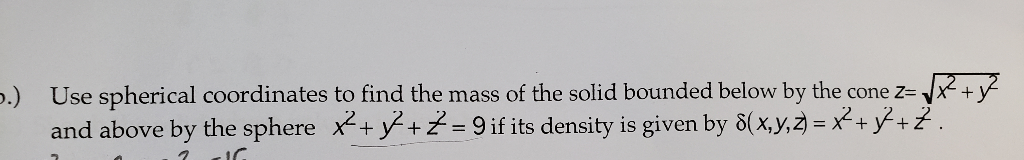Find the volume of the solid Use spherical coordinates to find the mass of the solid bounded below by the cone z=« .) and above by the sphere x+y+ =9if its density is given by 8(x,y,2) = x+ y+Z. JC Use spherical coordinates to find the mass of the solid bounded below by the cone z=« .) and above by the sphere x+y+ =9if its density is given by 8(x,y,2) = x+ y+Z. JC

• ### Find the volume of the given solid region bounded below by the cone z = \x²...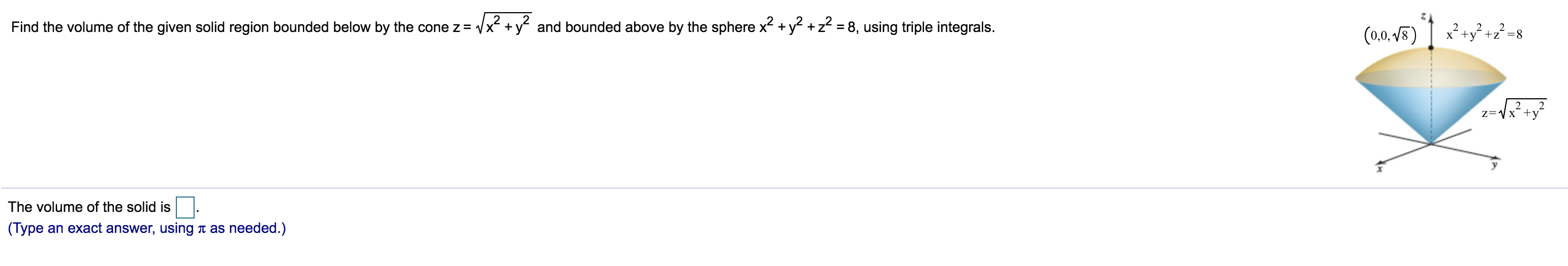Find the volume of the given solid region bounded below by the cone z = \x² + y2 and bounded above by the sphere x2 + y2 + z2 = 8, using triple integrals. (0,0,18) 5) 1 x? +y? +22=8 2-\x?+y? The volume of the solid is (Type an exact answer, using a as needed.)

• ### Evaluate the integral

Evaluate the triple integral in cylindrical coordinates to find the volume of the solid bounded above by the cone z=sqrt(x^2+y^2) and bounded below by the paraboloid,z=x^2+y^2.

Free Homework App